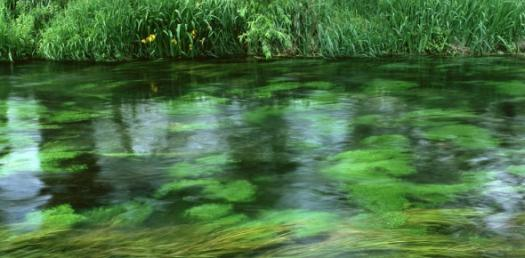# Nature Of Matter Quiz

25 Questions | Total Attempts: 1617SettingsCreate your own QuizNature of Matter

• 1.
Chemistry is the study of matter and how it changes.
• A.

True

• B.

False

• 2.
The two types of pure substances are elements and compounds.
• A.

True

• B.

False

• 3.
Light is matter.
• A.

True

• B.

False

• 4.
An atom is the smallest particle of matter that has the properties of a particular element.
• A.

True

• B.

False

• 5.
Molecules join together to form atoms.
• A.

True

• B.

False

• 6.
Energy is the ability to change or move matter.
• A.

True

• B.

False

• 7.
The Law of Conservation of Mass states that mass CAN be created and destroyed.
• A.

True

• B.

False

• 8.
The Law of Conservation of Energy states that energy CAN be created and destroyed.
• A.

True

• B.

False

• 9.
There are only 3 states of matter.
• A.

True

• B.

False

• 10.
Liquids have a fixed volume and fixed shape.
• A.

True

• B.

False

• 11.
Solids have a fixed volume and shape.
• A.

True

• B.

False

• 12.
Gases have a variable volume and shape.
• A.

True

• B.

False

• 13.
The viscosity of a fluid is its resistance to flow.
• A.

True

• B.

False

• 14.
The Kinetic Theory states that all matter is made of large items that are always still.
• A.

True

• B.

False

• 15.
A piece of tin has a mass of 16.52 g and a volume of 2.26 cubic centimeters.  What is the density of the tin?
• A.

D= 7.31 g/cubic centimeter

• B.

D= .731 g/cubic centimeter

• C.

D= 731 g/cubic centimeter

• D.

D= 7310 g/cubic centimeter

• 16.
What is the mass of an object that has a density of 8 g/per cubic centimeter and a volume of 64 cubic centimeters?
• A.

M= 8 g/per cubic centimeter

• B.

M= 50 g

• C.

M= 500 g

• D.

M= 64 cubic centimeters

• 17.
What is the volume of 325 g of metal with a density of 9 g/cubic centimeters?
• A.

V= 325 g

• B.

V= 36 cubic centimeters

• C.

V= 9 g/cubic centimeters

• D.

V= 360 cubic centimeters

• 18.
The formula for density is: Density equals mass over volume
• A.

True

• B.

False

• 19.
Chemical properties can be observed when one substance reacts with another.
• A.

True

• B.

False

• 20.
The density of a substance is equal to its mass divided by its volume.
• A.

True

• B.

False

• 21.
Physical properties can be observed or measured without changing the composition of matter.
• A.

True

• B.

False

• 22.
Changes of state, including melting, subliming, evaporating, boiling, condensing and freezing, are physical changes.
• A.

True

• B.

False

• 23.
Anything that has mass and takes up space is the definition of space.
• A.

True

• B.

False

• 24.
Na, Sodium, is a pure substance.
• A.

True

• B.

False

• 25.
Fe, Iron, is a pure substance.
• A.

True

• B.

False

Related TopicsBack to top
×

Wait!
Here's an interesting quiz for you.